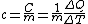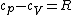# specific heat

Also found in: Dictionary, Thesaurus, Medical, Acronyms, Wikipedia.

## specific heat,

ratio of the heat capacityheat capacity
or thermal capacity,
ratio of the change in heat energy of a unit mass of a substance to the change in temperature of the substance; like its melting point or boiling point, the heat capacity is a characteristic of a substance.
..... Click the link for more information.
of a substance to the heat capacity of a reference substance, usually water. Heat capacity is the amount of heatheat,
nonmechanical energy in transit, associated with differences in temperature between a system and its surroundings or between parts of the same system. Measures of Heat
..... Click the link for more information.
needed to change the temperature of a unit mass 1°. The heat capacity of water is 1 calorie per gram per degree Celsius (1 cal/g-°C;) or 1 British thermal unit per pound per degree Fahrenheit (1 Btu/lb-°F;). Thus, the specific heat of some other substance relative to water will be numerically equal to its heat capacity; for this reason, "specific heat" is often used when the heat capacity actually is meant. Because the heat capacities of most substances vary with changes in temperature, the temperatures of both the specified substance and the reference substance must be known in order to give a precise value for the specific heat. The heat capacity of water at 15°C; is a frequently used value. Like specific gravity, specific heat is a dimensionless quantity, i.e., a pure number having no unit of measurement associated with it.
The Columbia Electronic Encyclopedia™ Copyright © 2013, Columbia University Press. Licensed from Columbia University Press. All rights reserved. www.cc.columbia.edu/cu/cup/

## Specific heat

A measure of the heat required to raise the temperature of a substance. When the heat ΔQ is added to a body of mass m, raising its temperature by ΔT, the ratio C given in Eq. (1) is defined as the heat capacity of the body. The quantity c defined in Eq. (2) is

(1)(2)called the specific heat capacity or specific heat. A commonly used unit for heat capacity is joule · kelvin-1 ( J · K-1); for specific heat capacity, the unit joule · gram-1 · K-1 ( J · g-1 · K-1) is often used. Joule should be preferred over the unit calorie = 4.18 J. As a unit of specific heat capacity, Btu · lb-1 · °F-1 = 4.21 J · g-1 · K-1 is also still in use in English-language engineering literature. If the heat capacity is referred to the amount of substance in the body, the molar heat capacity cm results, with the unit J · mol-1 · K-1.

If the volume of the body is kept constant as the energy ΔQ is added, the entire energy will go into raising its temperature. If, however, the body is kept at a constant pressure, it will change its volume, usually expanding as it is heated, thus converting some of the heat ΔQ into mechanical energy. Consequently, its temperature increase will be less than if the volume is kept constant. It is therefore necessary to distinguish between these two processes, which are identified with the subscripts V (constant volume) and p (constant pressure): CV, cV, and Cp, cp. For gases at low pressures, which obey the ideal gas law, the molar heat capacities differ by R, the molar gas constant, as given in Eq. (3), where R = 8.31 J · mol-1 · K-1; that is, the expanding gas heats up less.

(3)For solids, the difference between cpand cVis of the order of 1% of the specific heat capacities at room temperature. This small difference can often be ignored. See Heat capacity, Thermodynamic processes

McGraw-Hill Concise Encyclopedia of Physics. © 2002 by The McGraw-Hill Companies, Inc.

## specific heat

[spə′sif·ik ′hēt]
(thermodynamics)
The ratio of the amount of heat required to raise a mass of material 1 degree in temperature to the amount of heat required to raise an equal mass of a reference substance, usually water, 1 degree in temperature; both measurements are made at a reference temperature, usually at constant pressure or constant volume.
The quantity of heat required to raise a unit mass of homogeneous material one degree in temperature in a specified way; it is assumed that during the process no phase or chemical change occurs.
McGraw-Hill Dictionary of Scientific & Technical Terms, 6E, Copyright © 2003 by The McGraw-Hill Companies, Inc.

## specific heat

The ratio of the quantity of heat required to raise the temperature of a given mass of any substance 1 degree to the quantity required to raise the temperature of an equal mass of water 1 degree.
McGraw-Hill Dictionary of Architecture and Construction. Copyright © 2003 by McGraw-Hill Companies, Inc.
References in periodicals archive ?
The model predictions can be improved remarkably by considering the influences of polymer temperature on the density and specific heat capacity of polymer melt at constant pressure.
Specific Heat. It has been pointed out that the temperatures and water content have an important effect on specific heat [9,19], and Van Breugel  further proposes a calculation model of specific heat considering temperature, mixing ratio, and the hydration degree.
Caption: Figure 6: Specific heat versus temperature of water-based nanofluids loaded with various mass fractions of one-dimensional CuAl[O.sub.2] nanowires.
(2) The consideration of the influence of magnetic field upon the specific heat of [Er.sub.3]Ni shows that up to 1 T the regenerator would work satisfactorily.
where [gamma]T is the electronic specific heat term and [beta][T.sup.3] is the phonon specific heat term.
These differences result from the thermal conductivity and density and not the specific heat. In other words, the thermal conductivity is generally entered as a constant in the simulation, regardless of the temperature, while in reality the heat conductivity varies depending on temperature.
Specific heat values of the puller materials and rings (steel) have been adopted as for the temperature of 20 [degrees]C, due to the fact that charts on heats do not contain values for reference temperatures.
where [C.sub.pe] is effective specific heat, J/(kg x K), [phi] is porosity, [C.sub.pf] is specific heat of gas, J/(kg x K), and [C.sub.ps] is specific heat of solid, J/(kg x K).
1af) of the NaA zeolite and its cation exchanged samples, the thermodynamic parameters (enthalpy, entropy and specific heat), peak temperature, peak width, transition temperature, Debye velocity, Debye temperature, Debye wave vector, Fermi wave vector were calculated first and corresponding the most important the sizes of a- and AY-cages were calculated as listed in Table-2 and -3.
He said: "The surveyor found no evidence of specific heat loss but did point out that, as the garage is exposed on three sides and at roof level, it will cool at a quicker rate than the rest of his home.

Site: Follow: Share:
Open / Close